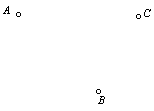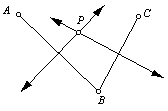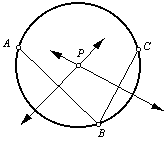Given three noncollinear points, construct the circle that includes all three points.

 1. Begin with points A, B, and C.2. Draw line segments AB and BC.3. Construct the perpendicular bisectors of line segments AB and BC. (Refer to the perpendicular bisector construction.) Let point P be the intersection of the perpendicular bisectors.4. Center the compass on point P, and draw the circle through points A, B, and C.Back to Geometry Construction Reference